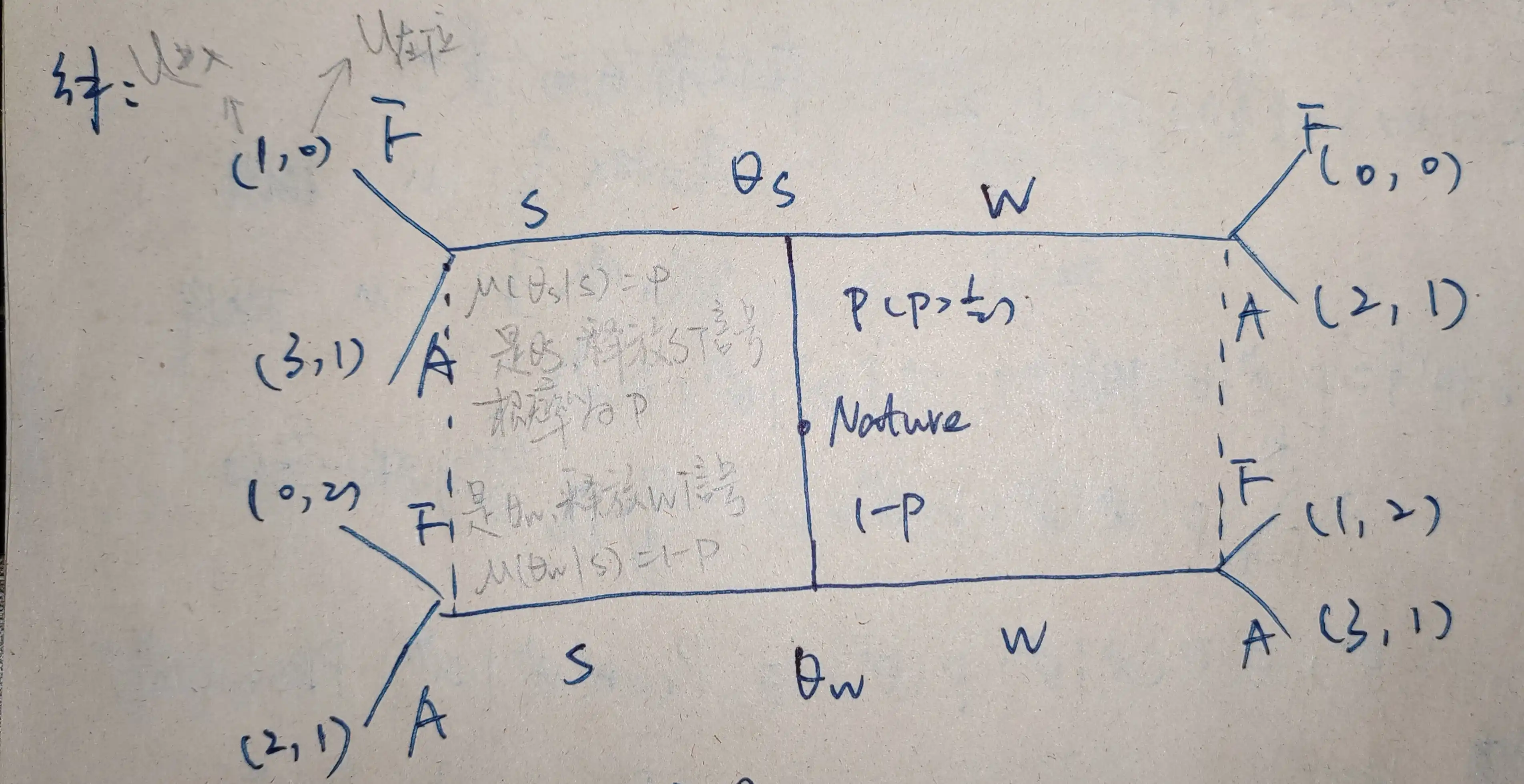# 【解】## (1) 考虑 $S_1=SW$。

\left\{\begin{matrix} \begin{aligned} \mu(\theta_s|S) &= 1, \\ \mu(\theta_w|W) &= 1. \end{aligned} \end{matrix} \right.

1) $p_1$ 类型为 $\theta_s$，释放 $S$ 信号， $p_2$ 选择 $A$ 的收益高； 2) $p_1$ 类型为 $\theta_w$，释放 $W$ 信号， $p_2$ 选择 $F$ 的收益高。

## (2) 考虑 $S_1=WS$。

\left\{\begin{matrix} \begin{aligned} \mu(\theta_s|S) &= 0, \\ \mu(\theta_s|W) &= 1. \end{aligned} \end{matrix} \right.

1) $p_1$ 类型为 $\theta_s$，释放 $W$ 信号， $p_2$ 选择 $A$ 的收益高； 2) $p_1$ 类型为 $\theta_w$，释放 $S$ 信号， $p_2$ 选择 $F$ 的收益高。

## (3) 考虑 $S_1=SS$。

\left\{\begin{matrix} \begin{aligned} \mu(\theta_s|S) &= P, \\ \mu(\theta_s|W) &= 任意值. \end{aligned} \end{matrix} \right.

$p_2$ 中，选 $A$ 的收益为 $B^{A}=1$，选 $F$ 的收益为 $B^{F}=0\times P+2\times(1-P)=2(1-P)$，

$P > 1/2.$

## (4) 考虑 $S_1=WW$。

\left\{\begin{matrix} \begin{aligned} \mu(\theta_s|w) &= P, \\ \mu(\theta_s|s) &= 任意值. \end{aligned} \end{matrix} \right.

$p_2$ 中，选 $A$ 的收益为 $B^{A}=1$，选 $F$ 的收益为 $B^{F}=0\times P+2\times(1-P)=2(1-P)$，

$P > 1/2.$